# MA.912.AR.5.7Export Print
Solve and graph mathematical and real-world problems that are modeled with exponential functions. Interpret key features and determine constraints in terms of the context.

### Examples

The graph of the function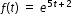can be transformed into the straight line y=5t+2 by taking the natural logarithm of the function’s outputs.

### Clarifications

Clarification 1: Key features are limited to domain; range; intercepts; intervals where the function is increasing, decreasing, positive or negative; constant percent rate of change; end behavior and asymptotes.

Clarification 2: Instruction includes representing the domain, range and constraints with inequality notation, interval notation or set-builder notation.

Clarification 3: Instruction includes understanding that when the logarithm of the dependent variable is taken and graphed, the exponential function will be transformed into a linear function.

Clarification 4: Within the Mathematics for Data and Financial Literacy course, problem types focus on money and business.

General Information
Subject Area: Mathematics (B.E.S.T.)
Strand: Algebraic Reasoning
Status: State Board Approved

## Benchmark Instructional Guide

### Terms from the K-12 Glossary

• Domain
• Exponent
• Exponential function
• Function
• Set-builder notation

### Vertical Alignment

Previous Benchmarks

Next Benchmarks

### Purpose and Instructional Strategies

In Algebra I, students worked with exponential functions in limited forms. In Math for Data and Financial Literacy, students solve problems modeled with exponential functions.
• Instruction focuses on real-world contexts that require students to create a function as a tool to determine requested information or should provide the group a function that models the context.
• For example, students can use exponential decay when analyzing the historical depreciation of a car. In MA.912.AR.2.5, students find the straight-line depreciation. In this benchmark, students could find the percentage the car depreciates instead of the dollar amount (exponential depreciation) using the equation $y$ = A(1 − $r$)$x$, where A is the starting value of the car, $r$ is the percent of depreciation, $x$ is the elapsed time in years, and $y$ is the value of the car after $x$ years.
• Instruction includes making the connection to logarithms when working with investments formulas involving exponents.
• For example, when using the formula A = P(1 + $\frac{\text{r}}{\text{n}}$)$n$$t$ where $r$ is the interest rate expressed as a decimal, $n$ is the number of times the interest is compounded annually, and $t$ is the term of the account, students may need to use logarithms to find the value of a variable.
• For example, when using the formula A = P$e$$r$$t$, students will need to use the natural logarithm to isolate the variables $r$ and $t$.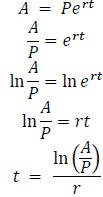• Instruction includes the use of technology and various representations of functions when solving problems. Given the complexity of the formulas in this course, it may be helpful for students to solve certain problems graphically rather than algebraically. Students can explore entering equations using graphing software.
• For example, if Sue deposited \$5,000 into an account that compounds interest monthly with an annual rate (APR) of 1.5%, students can determine how long it will take until the account reaches \$30,000 algebraically or graphically. To determine this graphically, students can graph the function $y$ = 5000(1 + $\frac{\text{0.015}}{\text{12}}$)12$t$ using technology. Students should realize that by finding where the graph reaches the $y$-value of 30,000, the corresponding $t$-value will provide how long it would take.
• For example, students can graph the equation 20000 =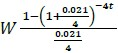to explore the relationship between the withdrawal amount and the time it takes to fully deplete a \$20,000 balance from an account that compounds quarterly at 2.1%.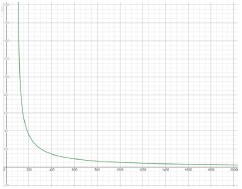• Problem types include using instances when periodic deposits are made into an account, and using formulas to find the term of systematic savings account. The formula to calculate the future value of a periodic deposit investment is B =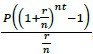, and the formula for the present value of a periodic deposit investment is P =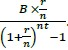.
• Problem types include using formulas to find the term of systematic withdrawals, which is when withdrawals are made at regular intervals. The formula to figure out the present value is P =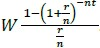.
• Be sure to highlight the relative ease and usefulness of graphing financial formulas to explore relationships. Graphs provide the visual data from an infinite number of calculations, saving students time and hassle when exploring relationships between variables.
• Instruction includes making connections to various forms of exponential functions to show their equivalency. Students should understand and interpret when solving the exponential in one form might be more useful than solving in another form depending on context.
• Instruction includes the use of $x$-$y$ notation and function notation.
• Instruction includes representing domain, range and intervals where the function is increasing, decreasing, positive or negative, using words, inequality notation, set-builder notation and interval notation.
• Words
If the domain is all real numbers, it can be written as “all real numbers” or “any value of $x$, such that $x$ is a real number."
• Inequality notation
If the domain is all values of $x$ greater than 2, it can be represented as $x$ > 2.
• Set-builder notation
If the range is all values of $y$ less than or equal to zero, it can be represented as {$y$|$y$ ≤ 0} and is read as “all values of $y$ such that $y$ is less than or equal to zero.”
• Interval notation
If the domain is all values of $x$ less than or equal to 3, it can be represented as (−∞, 3]. If the domain is all values of $x$ greater than 3, it can be represented as (3, ∞). If the range is all values greater than or equal to −1 but less than 5, it can be represented as [−1, 5).

### Common Misconceptions or Errors

• Ensure students are plugging the correct information into the formulas and using technology correctly. Grouping symbols on graphic software can be tricky. Model the entry of a few formulas with grouping symbols for students before releasing them to explore their own.
• Students may confuse finding the logarithm and natural logarithm.

• Luisa deposited \$15,000 into a savings account, which compounds interest monthly with an annual rate (APR) of 1.15%. Each month, she withdraws \$350 from this account to pay for travel expenses.
• Part A. How long will it take until the account is fully depleted?
• Part B. How can she change the amount she withdrawals so her account lasts twice as long?

• Han wants to set up a periodic investment account to save for a future car purchase. His goal is to save \$40,000. The best account he’s found pays a 2.3% annual interest rate, compounded monthly. Use the periodic investment formula below to graph a relationship between the amount of his monthly periodic investment, P, and the time it will take to achieve his goal, $t$.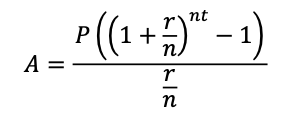### Instructional Items

Instructional Item 1
• Mindy purchased a 3-year-old car for \$22,300. When the car was new, it sold for \$35,500. Find the depreciation rate to the nearest hundredth of a percent.

*The strategies, tasks and items included in the B1G-M are examples and should not be considered comprehensive.

## Related Courses

This benchmark is part of these courses.
1200330: Algebra 2 (Specifically in versions: 2014 - 2015, 2015 - 2022, 2022 and beyond (current))
1200340: Algebra 2 Honors (Specifically in versions: 2014 - 2015, 2015 - 2022, 2022 and beyond (current))
1202340: Precalculus Honors (Specifically in versions: 2014 - 2015, 2015 - 2022, 2022 and beyond (current))
7912095: Access Algebra 2 (Specifically in versions: 2016 - 2018, 2018 - 2019, 2019 - 2022, 2022 and beyond (current))
1210305: Mathematics for College Statistics (Specifically in versions: 2022 and beyond (current))
1200388: Mathematics for Data and Financial Literacy Honors (Specifically in versions: 2022 and beyond (current))
1200384: Mathematics for Data and Financial Literacy (Specifically in versions: 2022 and beyond (current))
7912120: Access Mathematics for Data and Financial Literacy (Specifically in versions: 2022 - 2023, 2023 and beyond (current))
1200710: Mathematics for College Algebra (Specifically in versions: 2022 and beyond (current))
1209315: Mathematics for ACT and SAT (Specifically in versions: 2022 and beyond (current))

## Related Access Points

Alternate version of this benchmark for students with significant cognitive disabilities.
MA.912.AR.5.AP.7: Given a mathematical and/or real-world problem that is modeled with exponential functions, solve the mathematical problem, or select the graph using key features (in terms of context) that represents this model.

## Related Resources

Vetted resources educators can use to teach the concepts and skills in this benchmark.

## Formative Assessment

Interpreting Exponential Functions:

Students are asked to interpret parameters of an exponential function in context.

Type: Formative Assessment

## Lesson Plan

Which Function?:

This activity has students apply their knowledge to distinguish between numerical data that can be modeled in linear or exponential forms. Students will create mathematical models (graph, equation) that represent the data and compare these models in terms of the information they show and their limitations. Students will use the models to compute additional information to predict future outcomes and make conjectures based on these predictions.

Type: Lesson Plan

## Original Student Tutorials

Exponential Functions Part 3: Decay:

Learn about exponential decay as you calculate the value of used cars by examining equations, graphs, and tables in this interactive tutorial.

Type: Original Student Tutorial

Exponential Functions Part 2: Growth:

Learn about exponential growth in the context of interest earned as money is put in a savings account by examining equations, graphs, and tables in this interactive tutorial.

Type: Original Student Tutorial

Exponential Functions Part 1:

Learn about exponential functions and how they are different from linear functions by examining real world situations, their graphs and their tables in this interactive tutorial.

Type: Original Student Tutorial

## Perspectives Video: Professional/Enthusiast

KROS Pacific Ocean Kayak Journey: Kites, Wind, and Speed:

Lofty ideas about kites helped power a kayak from California to Hawaii.

Related Resources:
KROS Pacific Ocean Kayak Journey: GPS Data Set[.XLSX]
KROS Pacific Ocean Kayak Journey: Path Visualization for Google Earth[.KML]

Type: Perspectives Video: Professional/Enthusiast

## MFAS Formative Assessments

Interpreting Exponential Functions:

Students are asked to interpret parameters of an exponential function in context.

## Original Student Tutorials Mathematics - Grades 9-12

Exponential Functions Part 1:

Learn about exponential functions and how they are different from linear functions by examining real world situations, their graphs and their tables in this interactive tutorial.

Exponential Functions Part 2: Growth:

Learn about exponential growth in the context of interest earned as money is put in a savings account by examining equations, graphs, and tables in this interactive tutorial.

Exponential Functions Part 3: Decay:

Learn about exponential decay as you calculate the value of used cars by examining equations, graphs, and tables in this interactive tutorial.

## Student Resources

Vetted resources students can use to learn the concepts and skills in this benchmark.

## Original Student Tutorials

Exponential Functions Part 3: Decay:

Learn about exponential decay as you calculate the value of used cars by examining equations, graphs, and tables in this interactive tutorial.

Type: Original Student Tutorial

Exponential Functions Part 2: Growth:

Learn about exponential growth in the context of interest earned as money is put in a savings account by examining equations, graphs, and tables in this interactive tutorial.

Type: Original Student Tutorial

Exponential Functions Part 1:

Learn about exponential functions and how they are different from linear functions by examining real world situations, their graphs and their tables in this interactive tutorial.

Type: Original Student Tutorial

## Parent Resources

Vetted resources caregivers can use to help students learn the concepts and skills in this benchmark.